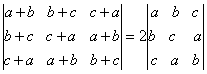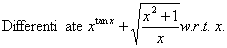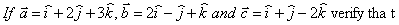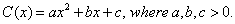Wednesday 14th April 2021

CBSE Guess > Papers > Question Papers > Class XII > 2006 > Mathematics > Compartment Outside Delhi Set - II.

Mathematics — 2006 (Set II — Compartment Outside Delhi)

SECTION – A

Q. 1. Using properties of determinants, prove the following:Q. 4. Two unbiased dice are thrown. Find the probability that the sum of the numbers obtained on the two dice is neither a multiple of 3 nor a multiple of 4.

Q. 10.Q. 14. Find the equation of the tangent to the curveQ. 18. Given the sum of the perimeters of a square and a circle, that the sum of their areas is least when the side of the square is equal to the diameter of the circle.

SECTION - B

Q. 20. IfQ. 21. A particle moves with uniform acceleration and passes 600 m in the 10th second and 720 m in the 12th second. Find the initial velocity of the particle.

Q. 25. Two forces acting at a point are such that if one of them is reversed the direction of the resultant is turned through a right angle. Prove that the two forces are equal in magnitude.

SECTION - C

Q. 20. A bill of Rs. 15,000 due on July 13, 2005 and another of Rs. 20,000 due on September 24, 2005 are both discounted on May 1, 2005. If the difference between the two discounts is Rs. 250, find the rate of discount.

Q. 21. Find the true discount and the banker’s gain on a bill for Rs. 15,500 due 3 months hence at 5% per annum.

Q. 22. A manufacturer of bulbs knows that on an average 5% of the bulbs are defective. He sells bulbs in packets of 100 each and guarantees that there will not be more than 4 defective bulbs in any packet. Find the probability of meeting the guarantee. (Use e-5-5 = 0.0067)

Q. 25. The cost of producing x units of a commodity isShow that the average cost and marginal cost are the same at the minimum average cost.

 Mathematics 2006 Question Papers Class XII Delhi Outside Delhi Compartment Delhi Compartment Outside DelhiSet ISet ISet ISet ISet IISet IISet IISet IISet IIISet III

 CBSE 2006 Question Papers Class XIIEnglishSociologyFunctional EnglishPsychologyMathematicsPhilosopyPhysicsComputer ScienceChemistryEntrepreneurshipBiologyInformatics PracticesGeographyMultimedia & Web TechnologyEconomicsBiotechnologyBusiness StudiesPhysical EducationAccountancyFine ArtsPolitical ScienceHistoryAgriculture